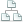Home >Tips >Excel 2016 from Scratch >Vertical lookup ASite map

# Excel 2016 from Scratch Exercise Vertical Lookup A

## An Excel tutorial by Peter KalmstromIn a recent tutorial in the Excel 2016 from Scratch series, Peter Kalmstrom has introduced formulas with the vertical lookup function. Here is an exercise, that you can try yourself before you look at the solution in the demo.

As an example Peter uses a campaign with discounts depending on how much you buy. The more you buy for, the bigger percentage of discount you get. The lookup formula finds to correct price span for each purchase.

### Exercise

If you want to try the exercise yourself, please download the Excel file Peter uses in the demo!

### Content

This is what Peter shows in the demo below:

• How to name cells.
• How to write formulas for multiplication with absolute references to the named cells and fill other cells with them.
• How to write formulas for multiplication and addition with a relative references and fill other cells with them.
• How to apply number format to cell ranges.
• How to apply Cell Styles to differentiate cells with input values from cells with calculated values.
• How to write a formula with a vertical lookup.

Peter uses Excel 2016 for his demo, but the Excel calculation functions are the same for earlier versions of Excel.